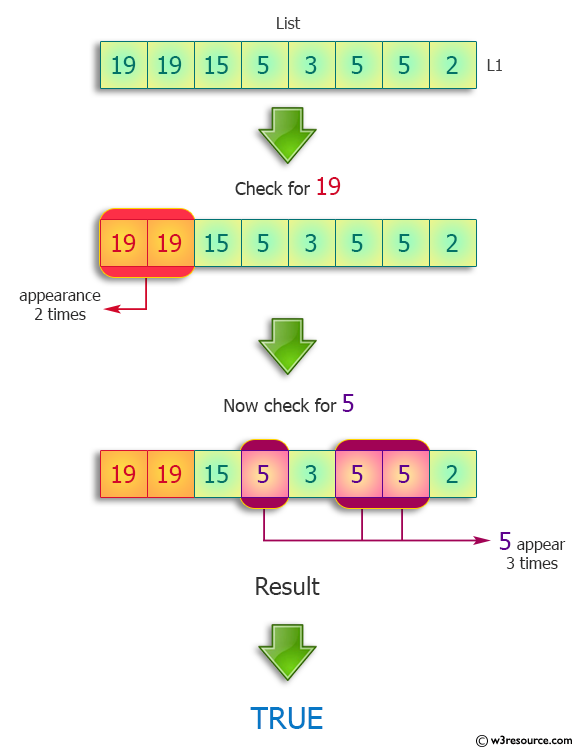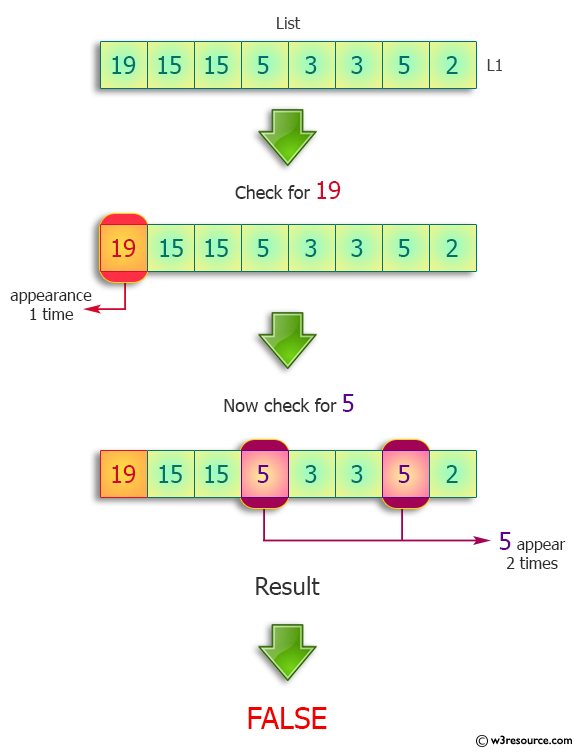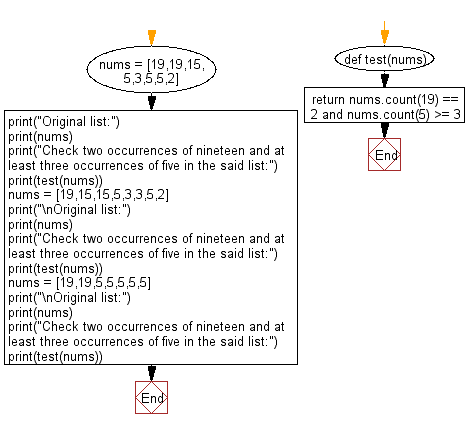﻿ Python: List of integers with exactly two occurrences of nineteen and at least three occurrences of five - w3resource# Python: List of integers with exactly two occurrences of nineteen and at least three occurrences of five

## Python Programming Puzzles: Exercise-1 with Solution

Write a Python program find a list of integers with exactly two occurrences of nineteen and at least three occurrences of five.

```Input:
[19, 19, 15, 5, 3, 5, 5, 2]
Output:
True

Input:
[19, 15, 15, 5, 3, 3, 5, 2]
Output:
False

Input:
[19, 19, 5, 5, 5, 5, 5]
Output:
True
```

Pictorial Presentation:Sample Solution:

Python Code:

``````#License: https://bit.ly/3oLErEI

def test(nums):
return nums.count(19) == 2 and nums.count(5) >= 3
nums = [19,19,15,5,3,5,5,2]
print("Original list:")
print(nums)
print("Check two occurrences of nineteen and at least three occurrences of five in the said list:")
print(test(nums))
nums = [19,15,15,5,3,3,5,2]
print("\nOriginal list:")
print(nums)
print("Check two occurrences of nineteen and at least three occurrences of five in the said list:")
print(test(nums))
nums = [19,19,5,5,5,5,5]
print("\nOriginal list:")
print(nums)
print("Check two occurrences of nineteen and at least three occurrences of five in the said list:")
print(test(nums))
``````

Sample Output:

```Original list:
[19, 19, 15, 5, 3, 5, 5, 2]
Check two occurrences of nineteen and at least three occurrences of five in the said list:
True

Original list:
[19, 15, 15, 5, 3, 3, 5, 2]
Check two occurrences of nineteen and at least three occurrences of five in the said list:
False

Original list:
[19, 19, 5, 5, 5, 5, 5]
Check two occurrences of nineteen and at least three occurrences of five in the said list:
True
```

Flowchart:## Visualize Python code execution:

The following tool visualize what the computer is doing step-by-step as it executes the said program:

Python Code Editor :

Have another way to solve this solution? Contribute your code (and comments) through Disqus.

What is the difficulty level of this exercise?

Test your Programming skills with w3resource's quiz.

﻿

## Python: Tips of the Day

Clamps num within the inclusive range specified by the boundary values x and y:

Example:

```def tips_clamp_num(num,x,y):
return max(min(num, max(x, y)), min(x, y))
print(tips_clamp_num(2, 4, 6))
print(tips_clamp_num(1, -1, -6))
```

Output:

```4
-1
```